Open in App
Not now

# GRE Geometry | Triangles

• Last Updated : 06 Sep, 2021

Triangle is one of the basic 2-D shapes in geometry. It consists of three angles, three side and three vertices, e.g.,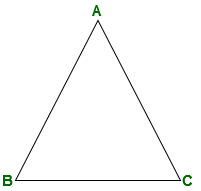On the basis of measurement of sides and angles there different types of triangle listed below:

1. Equilateral triangle:
All edges are equal, all angles are also equal to 60°, e.g.,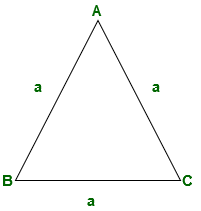2. Isosceles triangle:
Two edges are equal, hence two angles are also equal or sides opposite to equal sides are equal, e.g.,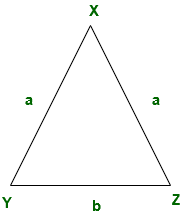3. Scalene triangle:
All edges are different, hence all angles are also different, e.g.,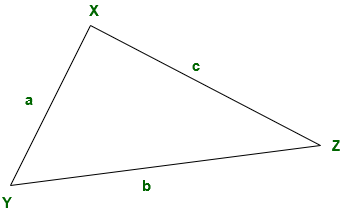4. Right angle triangle:
One angle is 90° and side opposite to 90° is the hypotenuse, e.g.,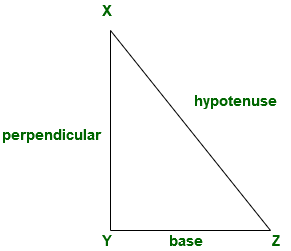5. Obtuse triangle:
One angle is obtuse, e.g.,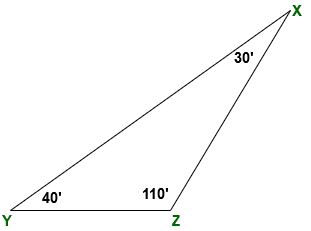6. Acute triangle:
All angles are acute, e.g.,Important properties of the triangle:

• Sum of angles of a triangle is 180°
• Third side of triangle is greater than the difference of other two sides and less than sum of other two sides.
• Area of triangle = (1 / 2) * base * height.
• In right angle triangle, hypotenuse2 = base2 + perpendicular2. [Pythagoras theorem]
• Area of equilateral triangle = (√3 / 4)side2

Congruency and similarity in triangles:

1. Congruent triangle:
Two triangles are congruent if shape and size of two triangles is same or two triangles have same angles and same sides, e.g.,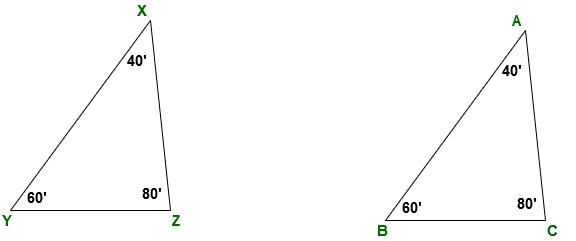Triangle ABC and XYZ are congruent.
or △ABC ≅△XYZ

Rules of congruency:

• (i) SSS (side – side – side) rule:
If all sides of a triangle is equal to all sides of another triangle. Then these two triangles are congruent to each other by SSS rule, e.g.,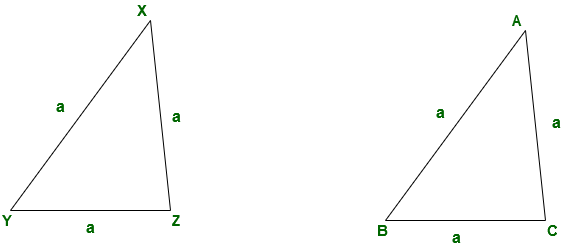• (ii) SAS (side – angle – side) rule:
If two sides of a triangle are equal to two sides in another triangle and angle between them is equal to angle between equal side in another triangle then these two triangles are congruent to each other by SAS rule, e.g.,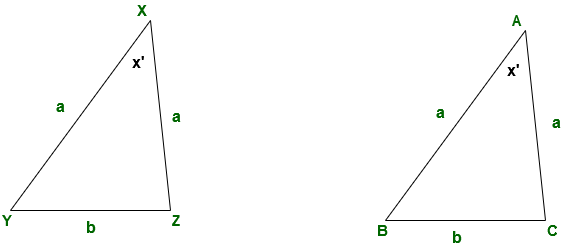• (iii) AAA (angle – angle – angle) rule:If all angles of a triangle are equal to all angles of another triangle then these two triangles are congruent to each other by AAA rule, e.g.,• (iv) ASA (angle – side – angle) rule:
If two angles of a triangle are equal to two angles in another triangle and side between them is equal to side between equal angles in another triangle then these two triangles are congruent to each other by ASA rule, e.g.,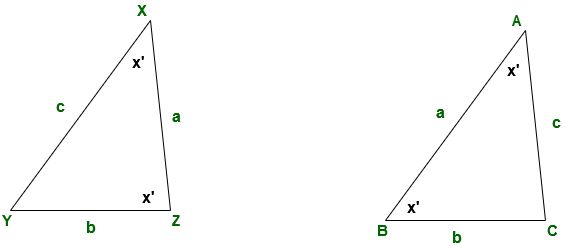• (v) AAS (angle – angle – side) rule:
If two angles and one side of a triangle is equal to two angles and one side in another triangle then these triangles are congruent to each other by AAS rule, e.g.,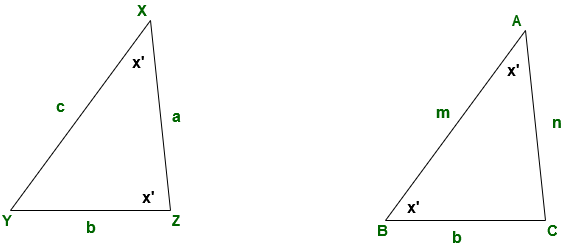2. Similar triangles:
Two triangles are similar if their shape is same but it is not necessary that their size is also same. To prove two triangles are similar scale factor of similarity is used, this factor check the equivalence of ratio of corresponding sides and corresponding angles, e.g.,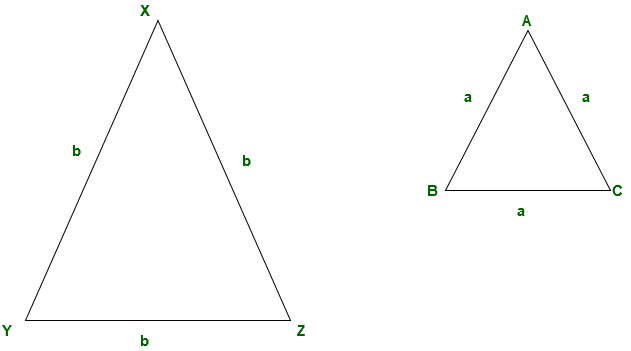`△ABC is similar to △XYZ. `

Since, △ABC is similar to △XYZ we can write scale factor of similarity as:

`XY / AB = XZ / AC = YZ / BC. `

To obtain AB/AC just cross multiply,

```XY / AB = XZ / AC.
AB / AC = XY / XZ.```
My Personal Notes arrow_drop_up
Related Articles